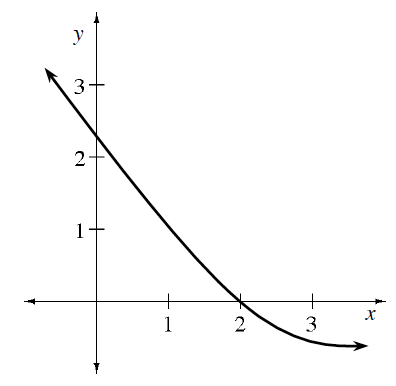### Home > APCALC > Chapter 11 > Lesson 11.4.1 > Problem11-116

11-116.

Multiple Choice: The graph of $y = f(x)$ is shown at right. The function is twice differentiable. Examine the graph and decide which statement below is true. Homework Help ✎

1. $f(2) < f ′(2) < f ″(2)$

2. $f(2) < f ″(2) < f ′(2)$

3. $f ′(2) < f(2) < f ″(2)$

4. $f ″(2) < f(2) < f ′(2)$

5. $f ″(2) < f ′(2) < f(2)$$f\left(2\right) = 0$
$f′(2) =$ slope at $x = 2$ (positive or negative?)
$f″\left(2\right) =$ concavity at $x = 2$ (up or down?)